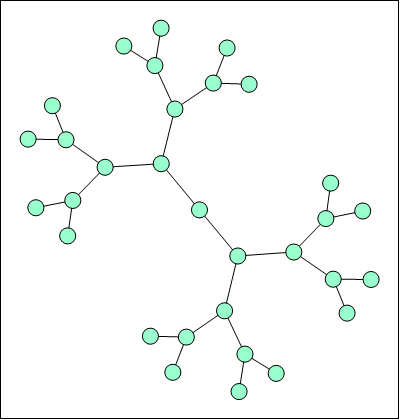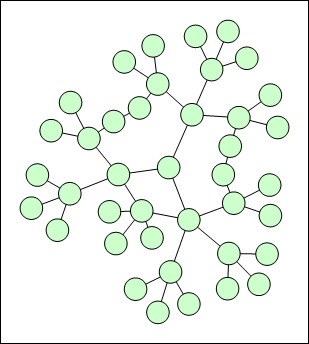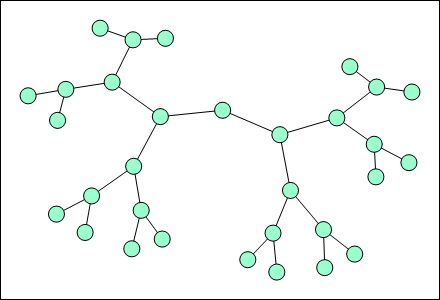﻿ Symmetrical Layout
Nevron .NET Vision
Diagram for .NET / User's Guide / Layouts / Graph Parts Layouts / Graph Layouts / Symmetrical Layout

In This Topic
Symmetrical Layout
In This Topic

The symmetrical layout is represented by the NSymmetricalLayout class. It represents an implementation of the Fruchertman and Reingold force directed layout (with some modifications). It uses attractive and repulsive forces, which aim to produce a drawing with uniform distance between each set of connected vertices. Because of that the drawing tends to be symmetrical.

The attractive and repulsive forces are coupled in an instance of the NDesiredDistanceForce class, accessible from the DesiredDistanceForce property. More precisely, the desired distance force is both a vertex force (acts on each pair of vertices) and an edge force (acts on each pair of vertices connected with an edge).

The vertex force component is always repulsive - its magnitude decreases in an inverse square law as the distance between the vertices increases.

The edge force component is always attractive - its magnitude increases in a square law as the distance between the vertices increases.

The two force components balance each other out at the desired distance specified by the DesiredDistance property of the force. Because of the square nature of the two force components the desired distance is approximately enforced almost through the entire graph. That is why graph drawings produced with this method tend to be symmetrical (with equal edge lengths).

The following image illustrates the result of the symmetrical layout:figure 1. Symmetrical layout used to layout a binary tree

Tuning Considerations

A major advantage of the symmetrical layout is that it is very easy to be tuned. The most common tuning is to specify the desired distance (length between the vertices centers):

C#
Copy Code
```// set the desired distance
symmetricalLayout.DesiredDistanceForce.DesiredDistance = 200;
```
Visual Basic
Copy Code
```' set the desired distance
symmetricalLayout.DesiredDistanceForce.DesiredDistance = 200
```

Another common consideration with the symmetrical layout is compactness. The layout can be easily compacted by enabling the gravity force:

C#
Copy Code
```// enable gravity force to increase compactness
symmetricalLayout.GravityForce.Enabled = true;
```
Visual Basic
Copy Code
```' enable gravity force to increase compactness
symmetricalLayout.GravityForce.Enabled = True
```

The following image illustrates the result of the symmetrical layout with enabled gravity:figure 2. Symmetrical layout with gravity force enabled

In rare cases when the graph admits a drawing with uniform edge direction you may also consider enabling the magnetic field force. The following example creates a magnetic field with parallel downward direction and unidirectional magnetization type:

C#
Copy Code
```// configure a magnetic field force
symmetricalLayout.MagneticFieldForce.Enabled = true;
symmetricalLayout.MagneticFieldForce.FieldDirection = MagneticFieldDirection.ParallelDownward;
symmetricalLayout.MagneticFieldForce.MagnetizationType = MagnetizationType.Unidirectional;
```
Visual Basic
Copy Code
```' configure a magnetic field force
symmetricalLayout.MagneticFieldForce.Enabled = True
symmetricalLayout.MagneticFieldForce.FieldDirection = MagneticFieldDirection.ParallelDownward
symmetricalLayout.MagneticFieldForce.MagnetizationType = MagnetizationType.Unidirectional
```

The following image illustrates the result of the symmetrical layout with disabled/enabled magnetic field:Figure 3. Normal symmetrical layout used to display a binary tree.Figure 4. Symmetrical layout with a parallel downward magnetic field and unidirectional edge magnetization.

Related Examples

Windows Forms: Layouts - Symmetrical Layout

Web Forms: Layouts - Symmetrical Layout